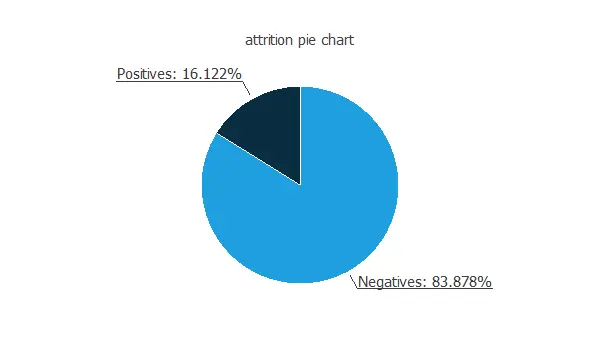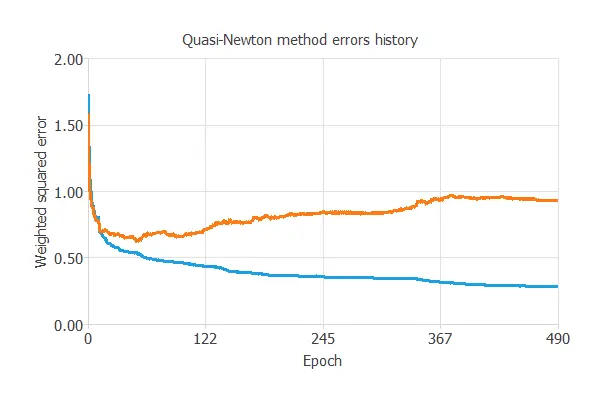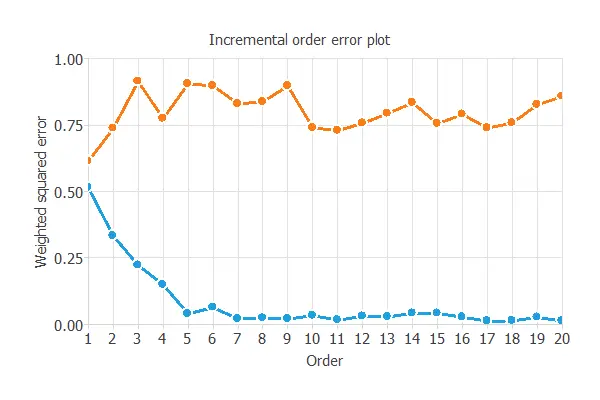﻿

Employee attrition prediction using machine learning

# Reduce employee attrition using Artificial IntelligenceOne of the main problems of companies and human resource departments is employee churn. This phenomenon can be costly since retaining an existing employee is far less expensive than acquiring a new one.

Employee churn prevention aims to predict who, when, and why employees will terminate their jobs.

Accurate methods that identify which employees are more likely to switch to another position are needed. These allow companies to change those specific aspects of the organization to prevent attrition and reduce costs.

The objective is to untangle all the factors that lead to employee attrition effectively and determine the underlying causes to prevent it.

### Contents:

This example is solved with Neural Designer. To follow it step by step, you can use the free trial.

## 1. Application type

This is a classification project, since the variable to be predicted is binary (attrition or not).

The goal here is to model the probability of attrition, conditioned on the employee features.

## 2. Data set

The data file employee_attrition.csv contains quantitative and qualitative information about a sample of employees at the company.

The data set contains about 1,500 employees (or instances). For each, around 35 personal, professional, and socio-economical attributes (or variables) are selected.

More specifically, the variables of this example are:

• Age.
• Business travel: Non-travel (0), rarely (1), frequently (2).
• Daily rate.
• Department: Sales, Research & Development, Human Resources.
• Distance from home.
• Education: 1, 2, 3, 4, 5.
• Education field: Life Sciences, Human Resources, Medical, Marketing, Technical Degree, Other.
• Employee count.
• Employee number.
• Environment satisfaction: 1, 2, 3, 4.
• Gender: Male, Female.
• Hourly rate.
• Job involvement: 1, 2, 3, 4.
• Job level: 1, 2, 3, 4, 5.
• Job role: Sales Executive, Research Scientist, Laboratory Technician, Manufacturing Director, Healthcare Representative, Manager, Sales Representative, Research Director, Human Resources.
• Job satisfaction: 1, 2, 3, 4.
• Marital status: Single, Divorced, Married.
• Monthly income.
• Monthly rate.
• Number of companies worked.
• Over 18: True or False.
• Over time: True or False.
• Percent salary hike.
• Performance rating: True or False.
• Relationship satisfaction: 1, 2, 3, 4.
• Standard hours: True or False.
• Stock option level: 0, 1, 2,3.
• Total working years.
• Training times last year.
• Work-life balance: 1, 2, 3, 4.
• Years at company.
• Years in current role.
• Years since last promotion.
• Years with current manager.
• Attrition: Loyal or Attrition.

We have 48 input variables, which contain the characteristics of every employee. On the other hand, we have 1 target variable, which is the variable “Attrition” mentioned before.

There are 3 constant variables (“EmployeeCount”, “Over18” and “StandardHours”). They will be set as unused variables for the analysis since they do not provide any valuable information.

Before starting the predictive analysis, it is important to know the distributions of the variables.

The following pie chart shows the ratio of negative and positive instances.The chart above shows that the data is unbalanced, i.e., the number of negative instances (1233) is much larger than the number of positive instances (237). We use this information later to design the predictive model properly.

The inputs-targets correlations analyze the dependencies between each input variable and the target.As we can see, the input variables that have more importance with the attrition are “OverTime” (0.246), “TotalWorkingYears” (0.223), and “YearsAtCompany” (0.196).

## 3. Neural network

The neural network takes all the employees’ attributes and will transform them into a probability of attrition.

For that purpose, we use a neural network composed of a scaling layer with 48 neurons, a perceptron layer with 3 neurons, and a probabilistic layer with 1 neuron.

## 4. Training strategy

The next step is to select an appropriate training strategy, which defines what the neural network will learn.

A general training strategy is composed of two concepts:

• A loss index.
• An optimization algorithm.

As we said before, the data set is unbalanced. Consequently, we set the weighted squared error as the error method, which assigns a weight to the positives instances of 5.20 and 1 to the negative instances. This makes the total weight for the positive instances equal to that for the negative instances.

We use the quasi-Newton method as the optimization algorithm.

Now, the model is ready to be trained. The following chart shows how the training and selection errors decrease with the epochs of the optimization algorithm.The final training and selection errors are training error = 0.285 WSE and selection error = 0.931 WSE, respectively.

## 5. Model selection

The objective of model selection is to find the network architecture with the best generalization properties, that is, that which minimizes the error on the selected instances of the data set.

More specifically, we want to find a neural network with a selection error of less than 0.931 WSE, which is the value that we have achieved so far.

Order selection algorithms train several network architectures with a different number of neurons and select that with the smallest selection error.

The incremental order method starts with a small number of neurons and increases the complexity at each iteration. The following chart shows the training error (blue) and the selection error (orange) as a function of the number of neurons.As we can see, the optimal number of neurons in the hidden layer is 1, resulting in an order selection error of 0.614 WSE, which is far better than the previous one.

## 6. Testing analysis

The testing analysis assesses the quality of the model to decide if it is ready to be used in the production phase, i.e., in a real-world situation.

The way to test the model is to compare the trained neural network’s outputs against the real targets for a set of data that has been used neither for training nor for selection, the testing subset. For that purpose, we make use of some testing methods commonly used in binary classification problems.

The ROC curve measures the discrimination capacity of the classifier between positives and negatives instances. For a perfect classifier, the ROC curve should pass through the upper left corner. The next chart shows the ROC curve of our problem.In this case, the area takes the value of 0.804, which confirms what we saw before in the ROC chart, that the model predicts attrition with high accuracy.

For classification models with a binary target variable, constructing the confusion matrix is also a good task to test the model. Below this table is displayed.

Predicted positive Predicted negative
Real positive 35 (11.9%) 16 (5.44%)
Real negative 47 (16%) 196 (66.7%)

The next list depicts the binary classification tests. They are calculated from the values of the confusion matrix.

• Classification accuracy: 78.6% (ratio of correctly classified samples).
• Error rate: 21.4% (ratio of misclassified samples).
• Sensitivity: 68.6% (percentage of actual positive classified as positive).
• Specificity: 80.6% (percentage of actual negative classified as negative).

In general, these binary classification tests show a good performance of the predictive model. Nevertheless, it is essential to highlight that this model has greater specificity than sensitivity, showing that it works better when detecting negative instances accurately.

### 7. Model deployment

Once we know that the model can accurately predict employee attrition, it can be used to evaluate employee satisfaction with the company. This is called model deployment.

The model takes the form of a function that takes an employee’s inputs and provides the predicted output. The mathematical expression, which is listed below, can be embedded into any software.

scaled_age = 2*(age-18)/(60-18)-1;
scaled_daily_rate = 2*(daily_rate-102)/(1499-102)-1;
scaled_Sales = 2*(Sales-0)/(1-0)-1;
scaled_Research&Development = 2*(Research&Development-0)/(1-0)-1;
scaled_HumanResources = 2*(HumanResources-0)/(1-0)-1;
scaled_distance_from_home = 2*(distance_from_home-1)/(29-1)-1;
scaled_education = 2*(education-1)/(5-1)-1;
scaled_LifeSciences = 2*(LifeSciences-0)/(1-0)-1;
scaled_Other = 2*(Other-0)/(1-0)-1;
scaled_Medical = 2*(Medical-0)/(1-0)-1;
scaled_Marketing = 2*(Marketing-0)/(1-0)-1;
scaled_TechnicalDegree = 2*(TechnicalDegree-0)/(1-0)-1;
scaled_HumanResources_1 = 2*(HumanResources_1-0)/(1-0)-1;
scaled_employee_number = 2*(employee_number-1)/(2068-1)-1;
scaled_environment_satisfaction = 2*(environment_satisfaction-1)/(4-1)-1;
scaled_gender = 2*(gender-0)/(1-0)-1;
scaled_hourly_rate = 2*(hourly_rate-30)/(100-30)-1;
scaled_job_involvement = 2*(job_involvement-1)/(4-1)-1;
scaled_job_level = 2*(job_level-1)/(5-1)-1;
scaled_SalesExecutive = 2*(SalesExecutive-0)/(1-0)-1;
scaled_ResearchScientist = 2*(ResearchScientist-0)/(1-0)-1;
scaled_LaboratoryTechnician = 2*(LaboratoryTechnician-0)/(1-0)-1;
scaled_ManufacturingDirector = 2*(ManufacturingDirector-0)/(1-0)-1;
scaled_HealthcareRepresentative = 2*(HealthcareRepresentative-0)/(1-0)-1;
scaled_Manager = 2*(Manager-0)/(1-0)-1;
scaled_SalesRepresentative = 2*(SalesRepresentative-0)/(1-0)-1;
scaled_ResearchDirector = 2*(ResearchDirector-0)/(1-0)-1;
scaled_HumanResources_1 = 2*(HumanResources_1-0)/(1-0)-1;
scaled_job_satisfaction = 2*(job_satisfaction-1)/(4-1)-1;
scaled_Single = 2*(Single-0)/(1-0)-1;
scaled_Married = 2*(Married-0)/(1-0)-1;
scaled_Divorced = 2*(Divorced-0)/(1-0)-1;
scaled_monthly_income = 2*(monthly_income-1009)/(19999-1009)-1;
scaled_monthly_rate = 2*(monthly_rate-2094)/(26999-2094)-1;
scaled_num_companies_worked = 2*(num_companies_worked-0)/(9-0)-1;
scaled_over_time = 2*(over_time-0)/(1-0)-1;
scaled_percent_salary_hike = 2*(percent_salary_hike-11)/(25-11)-1;
scaled_performance_rating = 2*(performance_rating-3)/(4-3)-1;
scaled_relationship_satisfaction = 2*(relationship_satisfaction-1)/(4-1)-1;
scaled_stock_option_level = 2*(stock_option_level-0)/(3-0)-1;
scaled_total_working_years = 2*(total_working_years-0)/(40-0)-1;
scaled_training_times_last_year = 2*(training_times_last_year-0)/(6-0)-1;
scaled_work_life_balance = 2*(work_life_balance-1)/(4-1)-1;
scaled_years_at_company = 2*(years_at_company-0)/(40-0)-1;
scaled_years_in_current_role = 2*(years_in_current_role-0)/(18-0)-1;
scaled_years_since_last_promotion = 2*(years_since_last_promotion-0)/(15-0)-1;
scaled_years_with_curr_manager = 2*(years_with_curr_manager-0)/(17-0)-1;
y_1_1 = Logistic (-0.132196+ (scaled_age*-1.61431)+ (scaled_business_travel*1.60471)+ (scaled_daily_rate*0.246393)+ (scaled_Sales*0.907402)+ (scaled_Research&Development*-0.517518)+ (scaled_HumanResources*0.313808)+ (scaled_distance_from_home*0.945042)+ (scaled_education*-0.754642)+ (scaled_LifeSciences*-0.577821)+ (scaled_Other*-0.498823)+ (scaled_Medical*-0.224641)+ (scaled_Marketing*0.118109)+ (scaled_TechnicalDegree*1.09709)+ (scaled_HumanResources_1*1.10928)+ (scaled_employee_number*0.50999)+ (scaled_environment_satisfaction*-1.21089)+ (scaled_gender*0.608194)+ (scaled_hourly_rate*0.414471)+ (scaled_job_involvement*-1.53346)+ (scaled_job_level*-1.14007)+ (scaled_SalesExecutive*0.108099)+ (scaled_ResearchScientist*1.39005)+ (scaled_LaboratoryTechnician*2.09738)+ (scaled_ManufacturingDirector*-1.39253)+ (scaled_HealthcareRepresentative*-0.342303)+ (scaled_Manager*-0.823216)+ (scaled_SalesRepresentative*1.33255)+ (scaled_ResearchDirector*-0.626333)+ (scaled_HumanResources_1*-0.0752408)+ (scaled_job_satisfaction*-1.37811)+ (scaled_Single*1.44477)+ (scaled_Married*-0.171722)+ (scaled_Divorced*-0.227707)+ (scaled_monthly_income*-1.23993)+ (scaled_monthly_rate*-0.106072)+ (scaled_num_companies_worked*1.49945)+ (scaled_over_time*2.04229)+ (scaled_percent_salary_hike*0.58747)+ (scaled_performance_rating*-0.4962)+ (scaled_relationship_satisfaction*-1.02995)+ (scaled_stock_option_level*-1.16647)+ (scaled_total_working_years*-0.232614)+ (scaled_training_times_last_year*-0.385595)+ (scaled_work_life_balance*-1.80506)+ (scaled_years_at_company*-0.778416)+ (scaled_years_in_current_role*-0.59614)+ (scaled_years_since_last_promotion*4.21654)+ (scaled_years_with_curr_manager*-3.53303));
non_probabilistic_attrition = Logistic (-1.78806+ (y_1_1*4.59128));
attrition = probability(non_probabilistic_attrition);

logistic(x){
return 1/(1+exp(-x))
}

probability(x){
if x < 0
return 0
else if x > 1
return 1
else
return x
}


Using the predictive model, we can simulate different scenarios and find the more significant factors for the attrition of a given employee. This information allows the company to act on those variables.